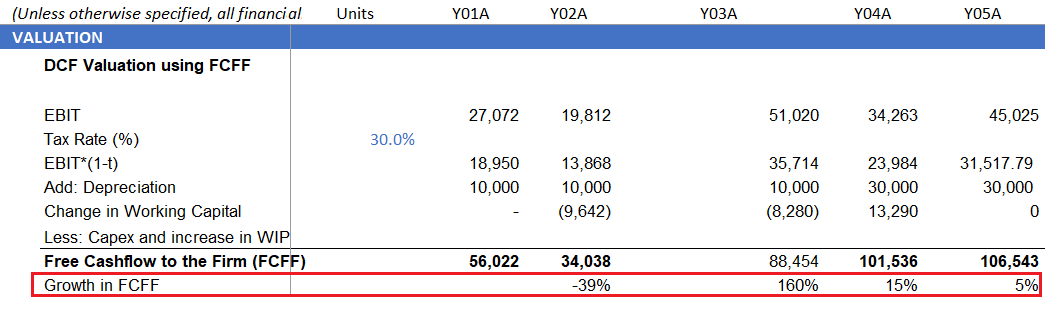•#### Hands-On Financial Modeling with Microsoft Excel 2019#### Overview of this book

Financial modeling is a core skill required by anyone who wants to build a career in finance. Hands-On Financial Modeling with Microsoft Excel 2019 explores terminologies of financial modeling with the help of Excel. This book will provides you with an overview of the steps you should follow to build an integrated financial model. You will explore the design principles, functions, and techniques of building models in a practical manner. Starting with the key concepts of Excel, such as formulas and functions, you will learn about referencing frameworks and other advanced components for building financial models. Later chapters will help you understand your financial projects, build assumptions, and analyze historical data to develop data-driven models and functional growth drivers. The book takes an intuitive approach to model testing and covers best practices and practical use cases. By the end of this book, you will have examined the data from various use cases, and have the skills you need to build financial models to extract the information required to make informed business decisions.
PrefaceFree Chapter
Section 1: Financial Modeling - OverviewIntroduction to Financial Modeling and ExcelSteps for Building a Financial ModelSection 2: The Use of Excel - Features and Functions for Financial ModelingFormulas and Functions - Completing Modeling Tasks with a Single FormulaApplying the Referencing Framework in ExcelSection 3: Building an Integrated Financial ModelUnderstanding Project and Building AssumptionsAsset and Debt SchedulesCash Flow StatementValuationRatio AnalysisModel Testing for Reasonableness and AccuracyAnother Book You May Enjoy# Creating a simple Monte Carlo simulation model

The Monte Carlo simulation is a model that calculates the probabilities of different results in a process where there is much inherent uncertainty. The model makes use of randomly generated numbers to obtain thousands of possible results, from which a most likely outcome can be deduced. We will look at growth in free cash flow, FCFF, as well as the cost of capital, WACC, which are both integral parts of our DCF model.

Calculate the FCFF growth rates for the historical years Y02A to Y05A using the following formula:The following screenshot shows the calculated historical growth in FCFF:Usually, Monte Carlo simulation uses thousands of repetitions. However, for illustration purposes, we will limit the number to 100. Let's take the average of FCFF historical growth.

The following screenshot shows the calculation of mean growth...